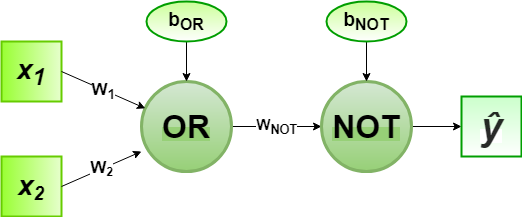# Implementation of Perceptron Algorithm for NOR Logic Gate with 2-bit Binary Input

• Last Updated : 08 Jul, 2020

In the field of Machine Learning, the Perceptron is a Supervised Learning Algorithm for binary classifiers. The Perceptron Model implements the following function:Attention reader! Don’t stop learning now. Get hold of all the important Machine Learning Concepts with the Machine Learning Foundation Course at a student-friendly price and become industry ready.

For a particular choice of the weight vectorand bias parameter, the model predicts outputfor the corresponding input vector.

NOR logical function truth table for 2-bit binary variables, i.e, the input vectorand the corresponding output001
010
100
110

We can observe that,Now for the corresponding weight vectorof the input vectorto the OR node, the associated Perceptron Function can be defined as:Later on, the output of OR nodeis the input to the NOT node with weight. Then the corresponding outputis the final output of the NOR logic function and the associated Perceptron Function can be defined as:For the implementation, considered weight parameters areand the bias parameters are.

Python Implementation:

 # importing Python libraryimport numpy as np  # define Unit Step Functiondef unitStep(v):    if v >= 0:        return 1    else:        return 0  # design Perceptron Modeldef perceptronModel(x, w, b):    v = np.dot(w, x) + b    y = unitStep(v)    return y  # NOT Logic Function# wNOT = -1, bNOT = 0.5def NOT_logicFunction(x):    wNOT = -1    bNOT = 0.5    return perceptronModel(x, wNOT, bNOT)  # OR Logic Function# w1 = 1, w2 = 1, bOR = -0.5def OR_logicFunction(x):    w = np.array([1, 1])    bOR = -0.5    return perceptronModel(x, w, bOR)  # NOR Logic Function# with OR and NOT  # function calls in sequencedef NOR_logicFunction(x):    output_OR = OR_logicFunction(x)    output_NOT = NOT_logicFunction(output_OR)    return output_NOT  # testing the Perceptron Modeltest1 = np.array([0, 1])test2 = np.array([1, 1])test3 = np.array([0, 0])test4 = np.array([1, 0])  print("NOR({}, {}) = {}".format(0, 1, NOR_logicFunction(test1)))print("NOR({}, {}) = {}".format(1, 1, NOR_logicFunction(test2)))print("NOR({}, {}) = {}".format(0, 0, NOR_logicFunction(test3)))print("NOR({}, {}) = {}".format(1, 0, NOR_logicFunction(test4)))
Output:
NOR(0, 1) = 0
NOR(1, 1) = 0
NOR(0, 0) = 1
NOR(1, 0) = 0


Here, the model predicted output () for each of the test inputs are exactly matched with the NOR logic gate conventional output () according to the truth table for 2-bit binary input.
Hence, it is verified that the perceptron algorithm for NOR logic gate is correctly implemented.

My Personal Notes arrow_drop_up